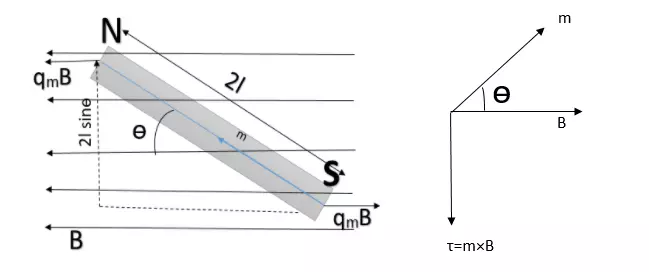# Torque on the magnetic dipole (bar magnet) in a uniform magnetic field, class 12

SHARING INCREASES KNOWLEDGE

In this article, we are going to discuss what if a bar magnet as a magnetic dipole is placed in a uniform magnetic field, so let’s get find out…

## Torque on the magnetic dipole in an uniform magnetic field

[latexpage]Let’s consider a bar magnet NS of length $2l$ placed in a uniform magnetic field $\vec{B}$. Let $q_{m}$ is the pole strength of each pole. Let the magnetic axis of the bar magnet makes an angle $\theta$ with a uniform magnetic field. See figure below:Fig. 1, (a). Torque on the bar magnet in a uniform magnetic field, (b). Relation between $\vec{\tau}$, $\vec{m}$ and $\vec{B}$, source: Vedantu.com

Force on North pole is $q_{m}B$, along $\vec{B}$, and force on South pole is $q_{m}B$ opposite to the $\vec{B}$.

We can see that the force on two pole is equal and opposite so they form a couple. The moment of couple or Torque is given as: $$\tau=\text{force}\times\text{perpendicular distance}$$ $$=q_{m}B\times 2l\sin\theta=(q_m\times 2l)B\sin\theta$$ or $$\boxed{\tau=mB\sin\theta}$$ where $m=q_m\times 2l$ is the magnetic dipole moment of the bar magnet. In vector notation, Torque can be given as: $$\vec{\tau}=\vec{m}\times \vec{B}$$

The direction of the torque $(\vec{\tau})$ can be given by the right-hand screw rule as indicated in the figure above (b).

### Effects of the torque on the bar magnet

The effects of the torque $\vec{\tau}$ on the bar magnet is to align the magnet itself in parallel to the external magnetic field $\vec{B}$ So that’s why a freely suspended magnet aligns itself in the North-South direction because the earth has its own magnetic field that exerts a torque on the magnet tending to align in the earth’s field direction (North-South direction).

### Special cases

1). When the magnet lies along the direction of the magnetic field.

θ = 0°, sin0° = 0, τ = 0

2). When the magnet lies perpendicular to the direction of the magnetic field.

θ = 90°, sin90° = 1, τ = mB

Thus, when θ is 90° (means magnet is perpendicular to the direction of the magnetic field) magnetic Torque is maximum. $$\tau_{max}= mB$$

### Definition of the magnetic dipole moment

If the magnetic field (B) is of unit strength and θ is 90°, then $\tau = m$. So the magnetic dipole moment can be defined as the torque acting on the magnetic dipole placed perpendicular to the uniform magnetic field having unit magnetic field strength.

#### SI unit of magnetic moment

From $\tau =mB\sin\theta$, magnetic moment can be given as: $$m=\frac{\tau}{B\sin\theta}$$ Therefore, SI unit of magnetic moment is: $$\text{unit of m}=\frac{1 Nm}{1 T.1}=NmT^{-1}=JT^{-1}=Am^2$$

Watch this video for more reference:

Stay tuned with Laws Of Nature for more useful and interesting content.

SHARING INCREASES KNOWLEDGE